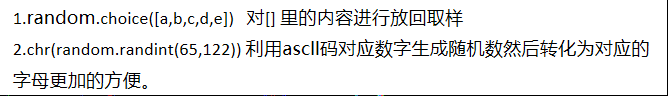﻿• 价格透明
• 信息保密
• 进度掌控
• 售后无忧# Python00001题：生成激活码

``````题目：#做为 Apple Store App 独立开发者，你要搞限时促销，为你的应用生成激活码（或者优惠券），使用 Python 如何生成 200 个激活码（或者优惠券）
``````
``````import random
a = []
for i in range(200):
n = ""
for m in range(15):
num = random.randint(0,9)
alf = chr(random.randint(65,122))
c = str(random.choice([num,alf])) #放回取样。
n += c
a.append(n)
``````3.通过这个小题了解更多的random库中的函数。### 低价透明### 金牌服务### 信息保密